Courses

# Number Representations MCQ Quiz - 1

## 20 Questions MCQ Test GATE Computer Science Engineering(CSE) 2022 Mock Test Series | Number Representations MCQ Quiz - 1

Description
This mock test of Number Representations MCQ Quiz - 1 for Computer Science Engineering (CSE) helps you for every Computer Science Engineering (CSE) entrance exam. This contains 20 Multiple Choice Questions for Computer Science Engineering (CSE) Number Representations MCQ Quiz - 1 (mcq) to study with solutions a complete question bank. The solved questions answers in this Number Representations MCQ Quiz - 1 quiz give you a good mix of easy questions and tough questions. Computer Science Engineering (CSE) students definitely take this Number Representations MCQ Quiz - 1 exercise for a better result in the exam. You can find other Number Representations MCQ Quiz - 1 extra questions, long questions & short questions for Computer Science Engineering (CSE) on EduRev as well by searching above.
QUESTION: 1

### What is the result of evaluating the following two expressions using three-digit floating point arithmetic with rounding? (113. + -111.) + 7.51 113. + (-111. + 7.51)

Solution: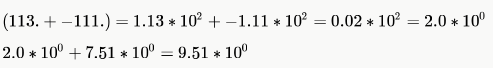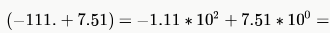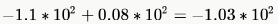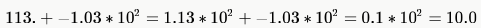QUESTION: 2

### Let A = 1111 1010 and B = 0000 1010 be two 8-bit 2’s complement numbers. Their product in 2’s complement is

Solution:

A = 1111 1010 = -6
B = 0000 1010 = 10
A*B = -60
=1100 0100

QUESTION: 3

### Using a 4-bit 2's complement arithmetic, which of the following additions will result in an overflow? i. 1100 + 1100 ii. 0011 + 0111 iii. 1111 + 0111

Solution:

Only (ii) is the answer.
In 2's complement arithmetic, overflow happens only when
1. Sign bit of two input numbers is 0, and the result has sign bit 1
2. Sign bit of two input numbers is 1, and the result has sign bit 0.
Overflow is important only for signed arithmetic while carry is important only for unsigned arithmetic.
A carry happens when there is a carry to (or borrow from) the most significant bit. Here, (i) and (iii) cause a carry but only (ii) causes overflow.

QUESTION: 4

The number (123456)8 is equivalent to

Solution:

(123456)8 = (001 010 011 100 101 110)2 = (00 1010 0111 0010 1110)2 = (A72E)16
= (00 10 10 01 11 00 10 11 10)2 = (22130232)4

QUESTION: 5

The range of integers that can be represented by an  bit 2’s complement number system is:

Solution:

An n-bit two's-complement numeral system can represent every integer in the range −(2n − 1) to +(2n − 1 − 1).
while ones' complement can only represent integers in the range −(2n − 1 − 1) to +(2n − 1 − 1).
A is answer

QUESTION: 6

The hexadecimal representation of 6578 is:

Solution:

6578 = (110 101 111)2 = (1 [10 10] [1 111])2 =(1AF)16

QUESTION: 7

We consider the addition of two 2`s complement numbers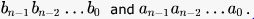A binary adder for adding unsigned binary numbers is used to add  the two numbers. The sum is denoted by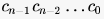and the carry-out byCout .
Which one of the following options correctly identifies the overflow condition?

Solution:

Number representation in 2's complement representation:

• Positive numbers as it is
• Negative numbers in 2's complement form.

So, the overflow conditions are
1. When we add two positive numbers (sign bit 0) and we get a sign bit 1
2. When we add two negative numbers (sign bit 1) and we get sign bit 0
3. Overflow is relevant only for signed numbers and we use carry for Unsigned numbers
4. When the carry out bit and the carry in to the most significant bit differs
PS: When we add one positive and one negative number we won't get a carry. Also points 1 and 2 is leading to point 4.
Now the question is a bit tricky. It is actually asking the condition of overflow of signed numbers when we use an adder
which is meant to work for unsigned numbers.
So, if we see the options, B is the correct one here as the first part takes care of case 2 (negative numbers) and the second part takes care of case 1 (positive numbers) - point 4.  We can see a counter example each for other options:

A - Let n = 4 and we do 0111 + 0111 =. This overflows as in 2`s complement representation we can store only up to 7. But the overflow condition in A returns false as .
Cout = 0.
C - This works for the above example. But fails for 1001 + 0001 = 1010 where there is no actual overflow (-7+1 = -6), but the given condition gives an overflow as Cout = 0 and cn-1 = 1.
D - This works for both the above examples, but fails for 1111 + 1111 = 1110 (-1 + -1 = -2) where there is no actual overflow but the given condition says so.

QUESTION: 8

The addition of 4-bit, two's complement, binary numbers 1101 and 0100 results in

Solution:

The addition results in 0001 and no overflow with 1 as carry bit.
In 2's complement addition Overflow happens only when :

• Sign bit of two input numbers is 0, and the result has sign bit 1.
• Sign bit of two input numbers is 1, and the result has sign bit 0.
QUESTION: 9

(C012.25)H - (10111001110.101)B =

Solution:

(C012.25)H - (10111001110.101)B
= 1100 0000 0001 0010. 0010 0101
-  0000 0101 1100 1110. 1010 0000
= 1011 1010 0100 0011. 1000 0101
= 1 011 101  001 000 011 . 100 001 010
= (135103.412)o
Binary subtraction is like decimal subtraction: 0-0 = 0, 1-1 = 0, 1-0 = 1, 0-1 = 1 with 1 borrow

QUESTION: 10

Let r denote number system radix. The only value(s) of r that satisfy the equation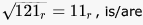Solution: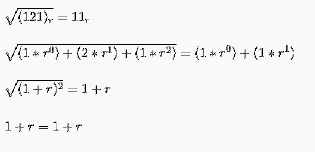So any integer r satisfies this but r must be > 2 as we have 2 in 121 and radix must be greater than any of the digits .(D) is the most appropriate answer

QUESTION: 11

A processor that has carry, overflow and sign flag bits as part of its program status word (PSW) performs addition of the following two 2's complement numbers 01001101 and 11101001. After the execution of this addition operation, the status of the carry, overflow and sign flags, respectively will be:

Solution:

01001101
+ 11101001
-----------------
100110110
Carry = 1
Overflow = 0 (In 2's complement addition Overflow happens only when : Sign bit of two input numbers is 0, and the result has sign bit 1 OR Sign bit of two input numbers is 1, and the result has sign bit 0.)
Sign bit = 0

QUESTION: 12

(1217)8 is equivalent to

Solution: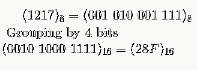QUESTION: 13

P is a 16-bit signed integer. The 2's complement representation of P is (F87B)16. The 2's complement representation of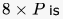Solution:

Multiplication can be directly carried in 2's complement form. F87B = 1111 1000 0111 1011 can be left shifted 3 times to give 8P = 1100 0011 1101 1000 = C3D8.
Or, we can do as follows:
MSB in (F87B) is 1. So, P is a negative number. So, P = -1 * 2's complement of (F87B) = -1 * (0785) = -1 * (0000 0111 1000 0101)
8 * P = -1 *  (0011 1100 0010 1000) (P in binary left shifted 3 times)
In 2's complement representation , this equals, 1100 0011 1101 1000 = C3D8

QUESTION: 14

The smallest integer that can be represented by an 8-bit number in 2's complement form is

Solution:

Range of 2's compliment no = > (- 2n-1)to + (2n-1 - 1)
Here n = No of bits = 8.
So minimum no = -2 ^ 7 = (B) -128

*Answer can only contain numeric values
QUESTION: 15

The base (or radix) of the number system such that the following equation holds is____________.  312/20 = 13.1

Solution:

Let ‘x’ be the base or radix of the number system .
The equation is : (3.x2+1.x1+2.x0) /(2.x1 +0.x0) =1.x1 +3.x0 +1.x-1
=>(3.x2+x +2) /(2.x) =x +3 +1/x
=>(3.x2+x +2) /(2.x) =(x2 +3x +1) /x
By solving above quadratic equation you will get  x=0 and x=5
As base or radix of a number system cannot be zero, here x = 5

*Answer can only contain numeric values
QUESTION: 16

Consider the equation (123)5 = (x8)y with x and y as unknown. THe number  of  possible solutions is ______.

Solution:

Changing (123) base 5 into base 10= 1*25+2*5+3*1=38

Changing x8 base y in decimal= x*y+8

Equating both we get xy+8=38

xy=30

possible combinations =(1,30)(2,15),(3,10)

but we have ‘8’ present in x8 so base y>8 as all three are satisfying the conditions so total solutions =3

*Answer can only contain numeric values
QUESTION: 17

Consider the equation (43)x = (y3)8 where x and y are unknow. The number of possible solutions is _____

Solution:

(43)x = (y3)8

Since a number in base -k can only have digits from 0 to (k-1), we can conclude that: x > 5 and y < 7
Now, the original equation, when converted to decimal base gives: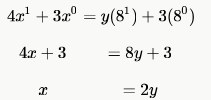So, we have the following constraints: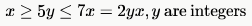The set of values of (x,y) that satisfy these constraints are: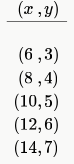I am counting 5 pairs of values.

QUESTION: 18

When two 8-bit numbers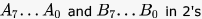complement representation (with A0 and B0 as the least significant bits) are added using a ripple-carry adder, the sum bits obtained are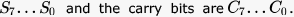and the carry bits are . An overflow is said to have occurred if

Solution:

Overflow is said to occur in the following cases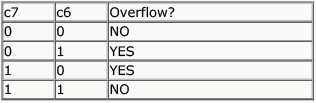The 3rd condition occurs in the following case A7B7S7', now the question arises how ?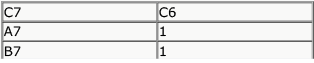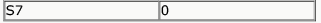NOW, A7=1 AND B7=1 S7=0 is only possible when C6=0 otherwise s7 would become 1 C7 has to be 1 (1+1+0 generates carry)
ON similar basis we can prove that C7=0 and C6=1 is produced by A7'B7'S7. Hence either of the two conditions cause overflow . Hence ans is C .
Why not A ? when C7=1 and C6 =1 this doesnt indicate overflow (4th row in the table)
Why not B ? if all carry bits are 1 then C7=1 and C6=1 (This also generates 4th row)
Why not D ? These combinations are C0 and C1 , the lower carrys dont indicate overflow

QUESTION: 19

The representation of the value of a 16 - bit unsigned integer X in hexadecimal number system is BC A9. The representation of the value of X in octal number system is

Solution:

Given: ( BCA9)16
1011 1100 1010 1001
....for octal number system...grouping of three- three bits from right to left..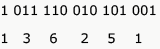Answer: Option D    (1 3 6  2 5 1)8

QUESTION: 20

A variable that takes thirteen possible values can be communicated using?

Solution:

As there are only 13 possible values variable can take, we can use ceil [log13] = 4 bits. As variable can take only 13 values we don't need to worry what those values are. Answer
:- Option D